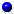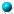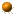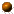Research Interests Dr. Michael MascagniComputational Science and Applications: Fast deterministic and stochastic numerical algorithms for computational materials science; computational biophysics; computational neuroscience; computational electrostatics; computational nanotechnologyComputer Science: Parallel and distributed computing system software; commodity-off-the-shelf cluster computing; cryptography and computer security; computational number theory; derandomization of probabilistic algorithms; probabilistic algorithms for quantum computersMathematical Biology: Numerical methods for neuronal modeling; reaction-diffusion equations; fast methods for branching nerve equations; particle methods; computational neuroscience; computational methods in biochemistryMonte Carlo Methods: Monte Carlo methods for partial differential equations; quasi-Monte Carlo methods for eigenproblems; first- and last-passage algorithms; applications to materials, biology, physics, and parallel computingNumerical Analysis: Deterministic particle and random gradient methods for reaction-diffusion equations; numerical integration; numerical linear algebra; graph partitioning; pseudo- and quasi random number generationParallel/Distributed/Grid Computing: Scalable pseudorandom number generation; scalable quasirandom number generation; parallel Monte Carlo and quasi-Monte Carlo methods; methods for parallel domain decomposition; validation of volunteered computation; Grid-based infrastructures for Monte CarloRandom Number Generation: Splitting and parameterization methods for parallel computing; SIMD and MIMD parallel testing and implementation, ASCI-class random numbers, quasirandom number generation; fast scrambling techniques quasirandom numbersSoftware Engineering: Scalable libraries for pseudorandom number generation, numerical libraries; efficient and portable implementations; scalable libraries for quasirandom number generation; robust distributed/Grid infrastructure for Monte Carlo applications; validation of Grid computing Home | Educational Background | Research Experience | Curriculum Vitae | Research Interests | Research Projects | Recent Papers | Courses | Abstracts of Talks | Brief Biography